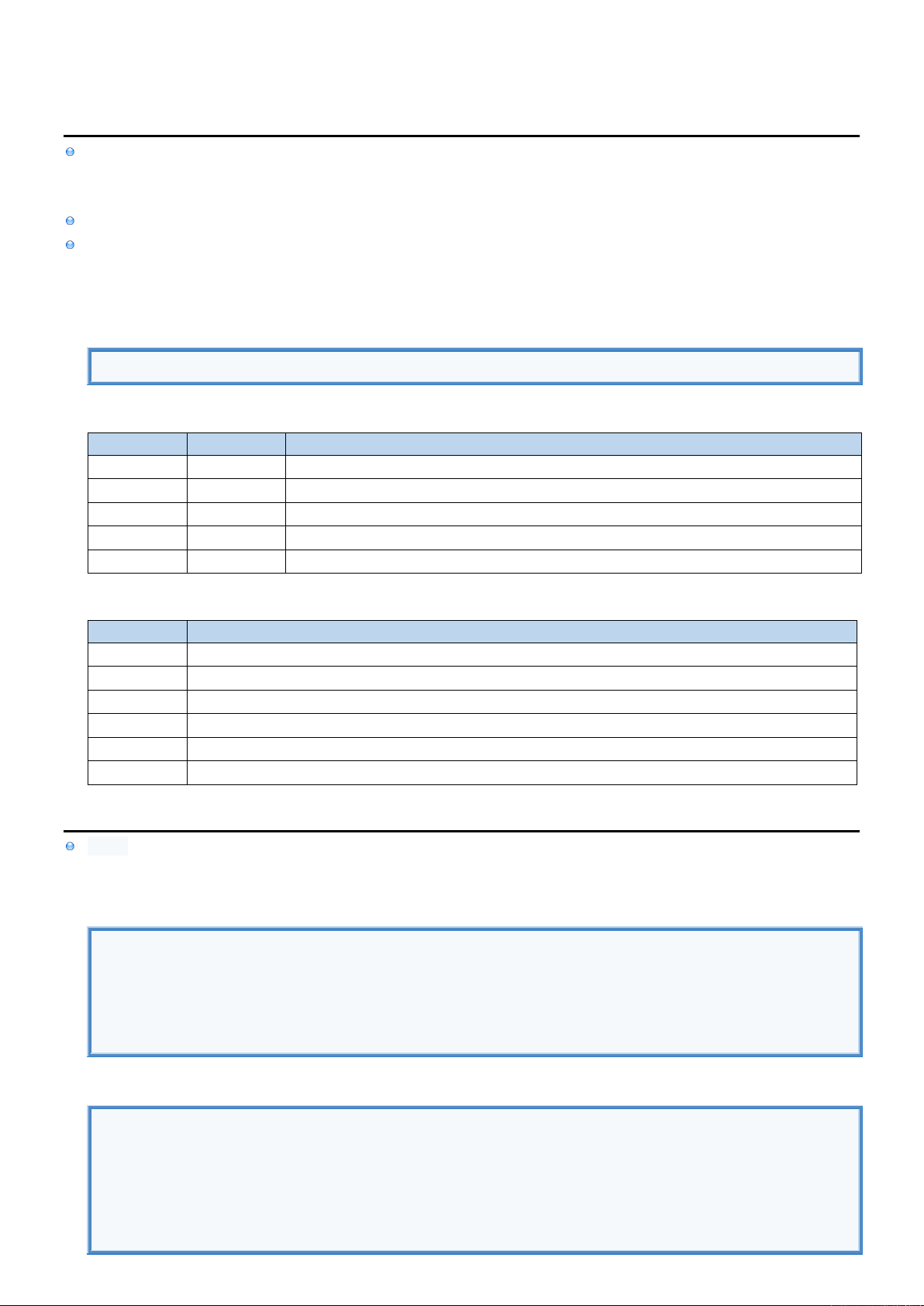2
2
.
.
4
4
.
.
7
7
P
P
a
a
t
t
t
t
e
e
r
r
n
n
s
s
I
I
n
n
f
f
o
o
[
[
R
R
]
]
[
[
R
R
]
]
[
[
R
R
]
]
Tuple Pattern
represents Tuple structure defining how menu Parameters should be in a Tuple and with what Values
is used to check if some Tuple Parameters have specific Value or to extract these Values
Single Values can be considered as Tuples with a single Parameter (in which case rounded brackets can be omitted).
Although Patterns represent a Tuple structure they can't be used to check if Tuple is of that Structure.
If Pattern represents Tuple with 2 elements you can only use it against the Tuple that also has 2 Parameters.
Otherwise check will throw an error if Tuple Pattern and Tuple Variable don't have the same structure.
Example
(x, 2, _) //Tuple Pattern that contains following other Patterns: Identifier, Value, Wildcard
Patterns
PATTERN
EXAMPLE
DESCRIPTION
Wildcard
"_"
We are not interested in the Value at this position
Value
"2"
Value at this position should be exactly 2
Range
"1...3"
Value at this position should be in the range [1, 3]
Identifier
"x"
Value at this position should be extracted into Variable "x" (if Tuple matches)
Tuple
"(x, 2)"
How many Values Tuple should have (use other Patterns inside the brackets)
Pattern Examples
NAME
DESCRIPTION
_
Tuple with 1 Value. Brackets can be omitted. Single Value Variable.
(_)
Tuple with 1 Value.
(_, _)
Tuple with 2 Values.
(_, 2)
Tuple with 2 Values. Second Value must be 2.
(_, 1...3)
Tuple with 2 Values. Second Value must be inside range [1, 3].
(x, 2, _)
Tuple with 3 Values. Second Value must be 2. If so first value is stored in Variable "x".
i
i
f
f
c
c
a
a
s
s
e
e
if case matches Tuple against the Patterns
(x, 2, _) is Tuple Pattern that contains following Patterns: Identifier, Value, Wildcard
Multiple Values Tuple
let point = (3, 2)
if case (_, _) = point { print("Tuple has 2 Parameters." ) }
if case (_, 2) = point { print("Tuple has 2 Parameters. Second Value is 2. ) }
if case (_, 1...3) = point { print("Tuple has 2 Parameters. Second Value is inside range [1, 3]." ) }
if case let (x, 2, _) = point { print("Tuple has 2 Parameters. Second Value is 2. First Value is \(x)." ) }
Single Value Tuple
let age = 20
if case (_) = age { print("Matched _" ) }
if case _ = age { print("Matched _" ) }
if case 20 = age { print("Matched 20" ) }
if case 1...30 = age { print("Matched 1...30") }
if case var x = age { print("Extracted \(x)") }f
f
o
o
r
r
l
l
o
o
o
o
p
p
Expression 1...3 creates range of Values (single Value Tuples).
For Loop cycles through range of Values (Tuples) and matches each Value against the Pattern
Wildcard Pattern "_"
Identifier Pattern "x"
for loop
for _ in 1...3 { print("Value is ignored") }
for x in 1...3 { print(x) } //Value is stored into variable "x"